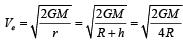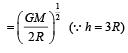Courses

# Test: Gravitation 1 - From Past 28 Years Questions

## 17 Questions MCQ Test Physics 28 Years Past year papers for NEET/AIPMT Class 11 | Test: Gravitation 1 - From Past 28 Years Questions

Description
This mock test of Test: Gravitation 1 - From Past 28 Years Questions for NEET helps you for every NEET entrance exam. This contains 17 Multiple Choice Questions for NEET Test: Gravitation 1 - From Past 28 Years Questions (mcq) to study with solutions a complete question bank. The solved questions answers in this Test: Gravitation 1 - From Past 28 Years Questions quiz give you a good mix of easy questions and tough questions. NEET students definitely take this Test: Gravitation 1 - From Past 28 Years Questions exercise for a better result in the exam. You can find other Test: Gravitation 1 - From Past 28 Years Questions extra questions, long questions & short questions for NEET on EduRev as well by searching above.
QUESTION: 1

### Two satellites of earth, S1 and S2 are moving in the same orbit. The mass of S1 is four times the mass of S2. Which one of the following statements is true? 

Solution:

Since orbital velocity of satellite is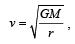it does not depend upon the mass of the satellite.
Therefore, both satellites will move with same speed.

QUESTION: 2

### A roller coaster is designed such that riders experience “weightlesness” as they go round the top of a hill whose radius of curvature is 20 m.The speed of the car at the top of the hill is between: 

Solution:

For the riders to experience weightlessness at the top of the hill, the weight of the radius must be balanced by the centripetal force.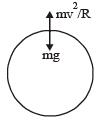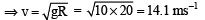Hence, the speed of the car should be between 14 ms–1 and 15 ms–1.

QUESTION: 3

### The figure shows elliptical or bit of a planet m about the sun S. The shaded area SCD is twice the shaded area SAB. If t1 is the time for the planet to move from C to D and t2 is the time to move from A to B then :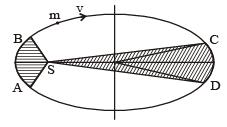Solution:

According to Kepler ’s law, the areal velocity of a planet around the sun always remains constant.
SCD : A1–  t1  (areal velocity constant)
SAB : A2 – t2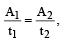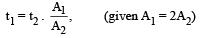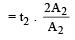∴   t1 = 2t2

QUESTION: 4

The radii of circular orbits of two satellites A and B of the earth, are 4R and R, respectively. If the speed of satellite A is 3 V, then the speed of satellite B will be: 

Solution:

Orbital velocity of a satellite in a circular orbit of radius a is given by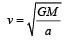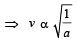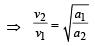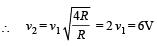QUESTION: 5

A particle of mass M is situated at the centre of a spherical shell of same mass and radius a. The gravitational potential at a point situated at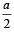distance from the centre, will be: 

Solution:

Potential at the given point = Potential at the point due to the shell + Potential due to the particle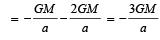QUESTION: 6

A planet moving along an elliptical orbit is closest to the sun at a distance r1 and farthest away at a distance of r2. If v1 and v2 are the linear velocities at these points respectively, then the ratio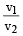is

Solution:

Angular momentum is conserved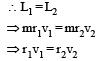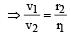QUESTION: 7

A particle of mass m is thrown upwards from the surface of the earth, with a velocity u. The mass and the radius of the earth are, respectively, M and R. G is gravitational constant and g is acceleration due to gravity on the surface of the earth. The minimum value of u so that the particle does not return back to earth, is [2011M]

Solution:

The velocity u should be equal to the escape velocity. That is,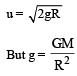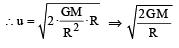QUESTION: 8

A particle of mass M is situated at the centre of spherical shell of mass M and radius a. The magnitude of the gravitational potential at a point situated at a/2 distance from the centre, will be [2011M]

Solution: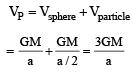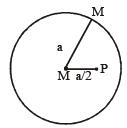QUESTION: 9

The height at which the weight of a body becomes 1/16th, its weight on the surface of earth (radius R), is : 

Solution:

Let at h height, the weight of a body becomes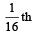of its weight on the surface.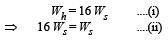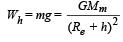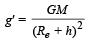Similarly,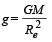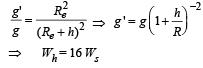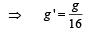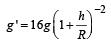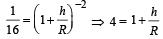h = 3R

QUESTION: 10

A spherical planet has a mass MP and diameter DP. A particle of mass m falling freely near the surface of this planet will experience an acceleration due to gravity, equal to :  

Solution:

Gravitational attraction force on particle B,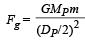Acceleration of particle due to gravity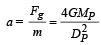QUESTION: 11

A geostationary satellite is orbiting the earth at a height of 5R above that surface of the earth, R being the radius of the earth. The time period of another satellite in hours at a height of 2R from the surface of the earth is : 

Solution:

According to Kelpner’s law of period

T2 ∝ R3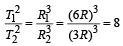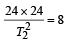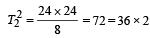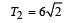QUESTION: 12

If ve is escape velocity and v0 is orbital velocity of a satellite for orbit close to the earth’s surface, then these are related by : [2012M]

Solution:

Escape velocity, ve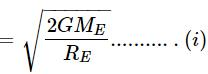Where Mg and RE be the mass and radius of the earth respectively. The orbital velocity of a satallite close to the earth's surface is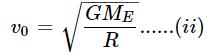From (i) and (ii), we get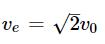QUESTION: 13

Which one of the following plots represents the variation of gravitational field on a particle with distance r due to a thin spherical shell of radius R ? (r is measured from the centre of the spherical shell) [2012M]

Solution:

The Gravitational field due to a thin spherical shell of radius R at distance r.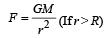For   r = R i.e. on the surface of the shell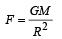For r < R  i.e. inside the shell

F = 0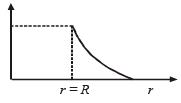QUESTION: 14

A body of mass ‘m’ is taken from the earth’s surface to the height equal to twice the radius (R) of the earth. The change in potential energy of body will be [NEET 2013]

Solution:

Initial P. E.,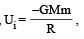Final P.E.,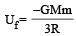[∵ R' = R + 2R = 3R]
∴ Change in potential energy,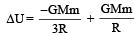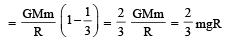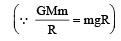ALTERNATE :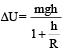By placing the value of h = 2R we get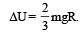QUESTION: 15

Infinite number of bodies, each of mass 2 kg are situated on x-axis at distances 1m, 2m, 4m, 8m, ..... respectively, from the origin. The resulting gravitational potential due to this system at the origin will be [NEET 2013]

Solution: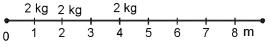Gravitational potential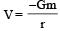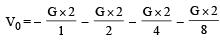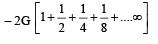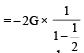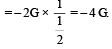QUESTION: 16

The radius of a planet is twice the radius of earth.Both have almost equal average mass-densities.If VP and VE are escape velocities of the planet and the earth, respectively, then [NEET Kar. 2013]

Solution:

Escape velocity,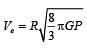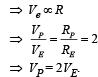QUESTION: 17

A particle of mass ‘m’ is kept at rest at a height 3R from the surface of earth, where ‘R’ is radius of earth and ‘M’ is mass of earth. The minimum speed with which it should be projected, so that it does not return back, is (g is acceleration due to gravity on the surface of earth) [NEET Kar. 2013]

Solution:

As we know, the minimum speed with which a body is projected so that it does not return back is called escape speed.